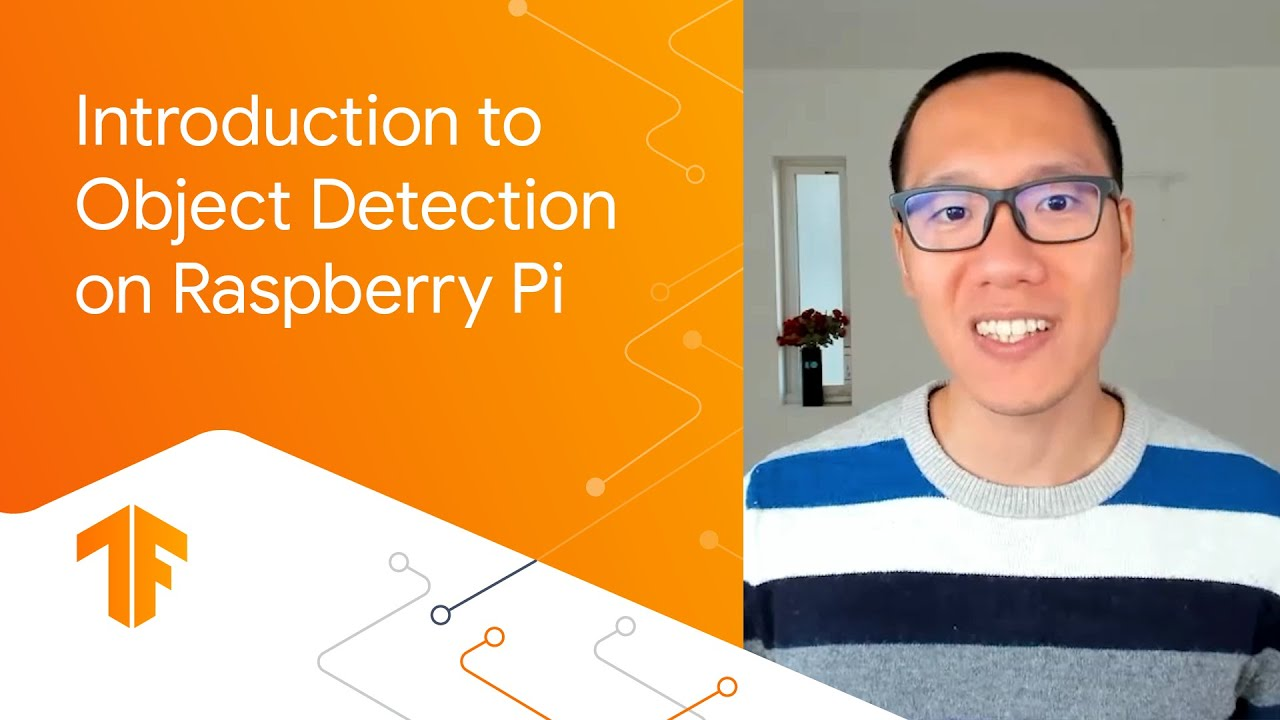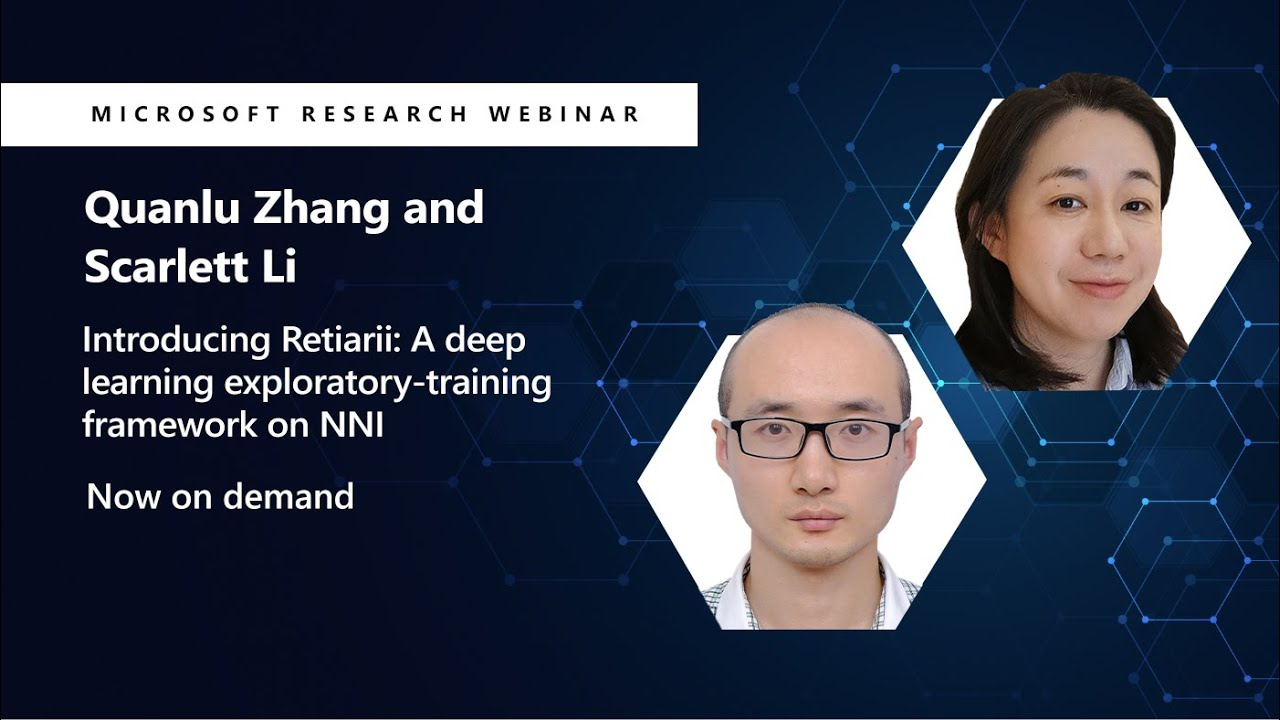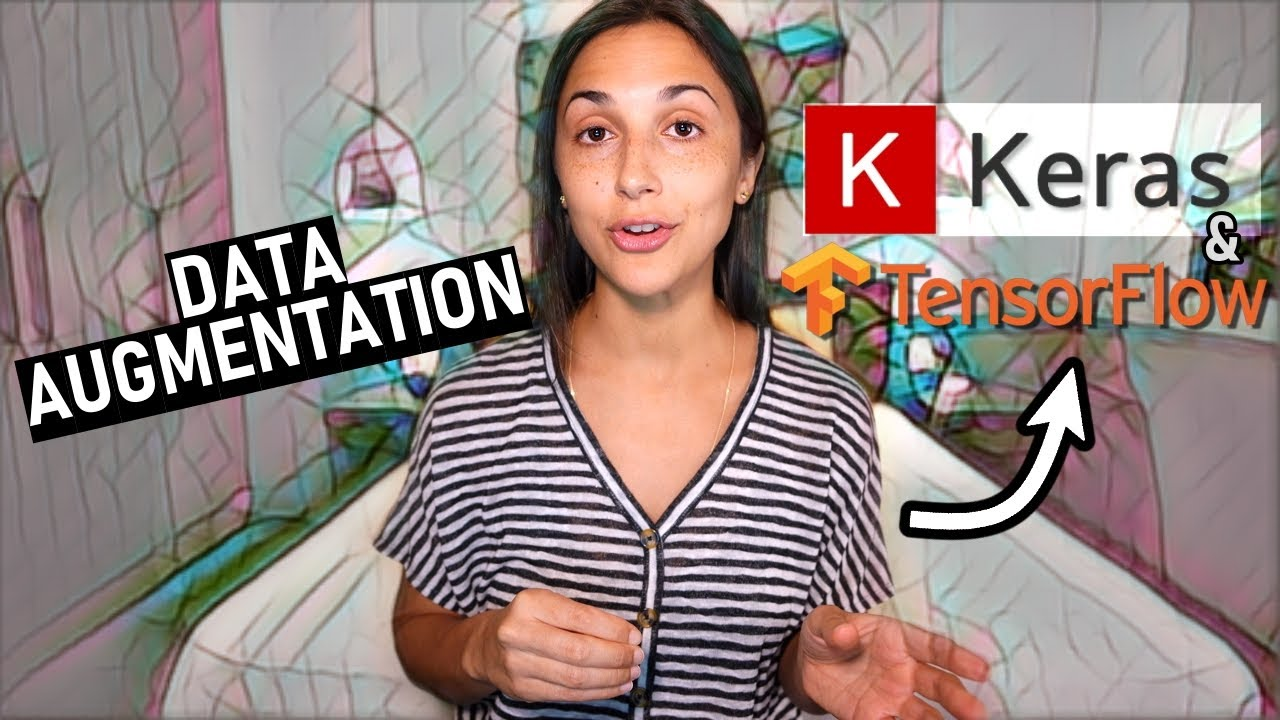TensorFlowComputer Vision Raspberry Pi TensorFlow

Introduction to object detection on Raspberry Pi

In the first episode of Machine Learning for Raspberry Pi, learn how to download a pre-trained TensorFlow Lite object detection model and deploy it to your Raspberry Pi. This model can be used to recognize general objects such as an apple, a television, or a car.TensorFlow

How to make TensorFlow models run faster on GPUs

XLA compilation on GPU can greatly boost the performance of your models, Learn how to use @tf.function(jit_compile=True) in TensorFlow to control what exact scopes are being compiled, and how to debug the performance of the resulting program. We’ll cover writing compiled models, debugging them, and exploring the performance characteristics and optimizations the XLA compiler performs, […]Generative AI

Intuitive Intro To Generative Adversarial Networks – GAN Course Preview

deeplizard  has a brand new premium course, an Introduction to Generative Adversarial Networks (GANs). Below is the full first lesson of the course available as a preview to the course at large.Generative AI

Intro to Generative Adversarial Networks (GANs) – New Course Release

deeplizard  announces their  brand new premium deeplizard course, an Introduction to Generative Adversarial Networks (GANs)AI Research

Introducing Retiarii: A deep learning exploratory-training framework on NNI

Traditional deep learning frameworks such as TensorFlow and PyTorch support training on a single deep neural network (DNN) model, which involves computing the weights iteratively for the DNN model. Designing a DNN model for a task remains an experimental science and is typically a practice of deep learning model exploration. Retrofitting such exploratory-training into the […]Deep Learning

Convolutions in Deep Learning – Interactive Demo App

deeplizard explains the importance of convolutions in deep learning. In deep learning, convolution operations are the key components used in convolutional neural networks. A convolution operation maps an input to an output using a filter and a sliding window. Use the interactive demonstration below to gain a better understanding of this process.Natural Language Processing TensorFlow

Use ML.NET to Score a Tensorflow Text Classification Model

Jon Wood recently streamed how to go over how to use a pre-trained TensorFlow model to predict sentiment within ML.NET.AI Natural Language Processing

Intelligent AI Chatbot in Python

In today’s video, NeuralNine going to build an intelligent AI chatbot using neural networks and natural language processing in Python.Quantum Computing

Quantum Computing Basics – Creating Qubits

YouTuber BharatiDWConsultancy provides a quick overview of quantum computing and its fundamental building block: the qubit!